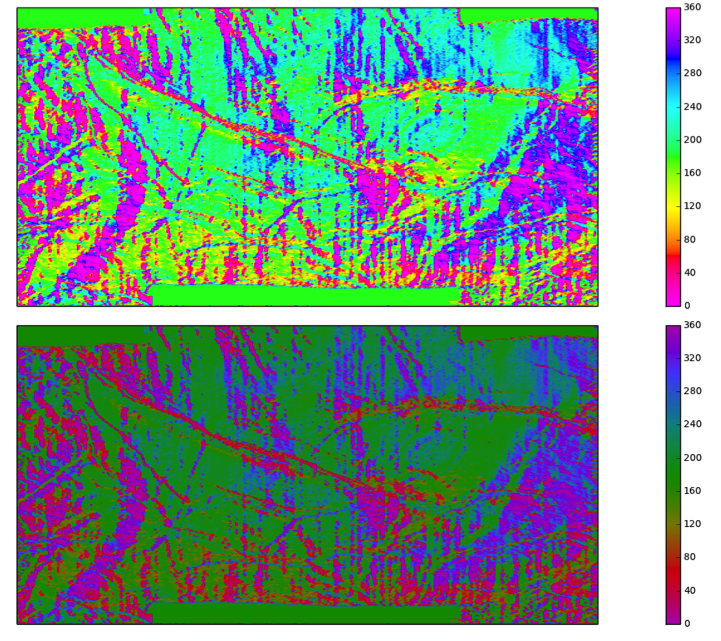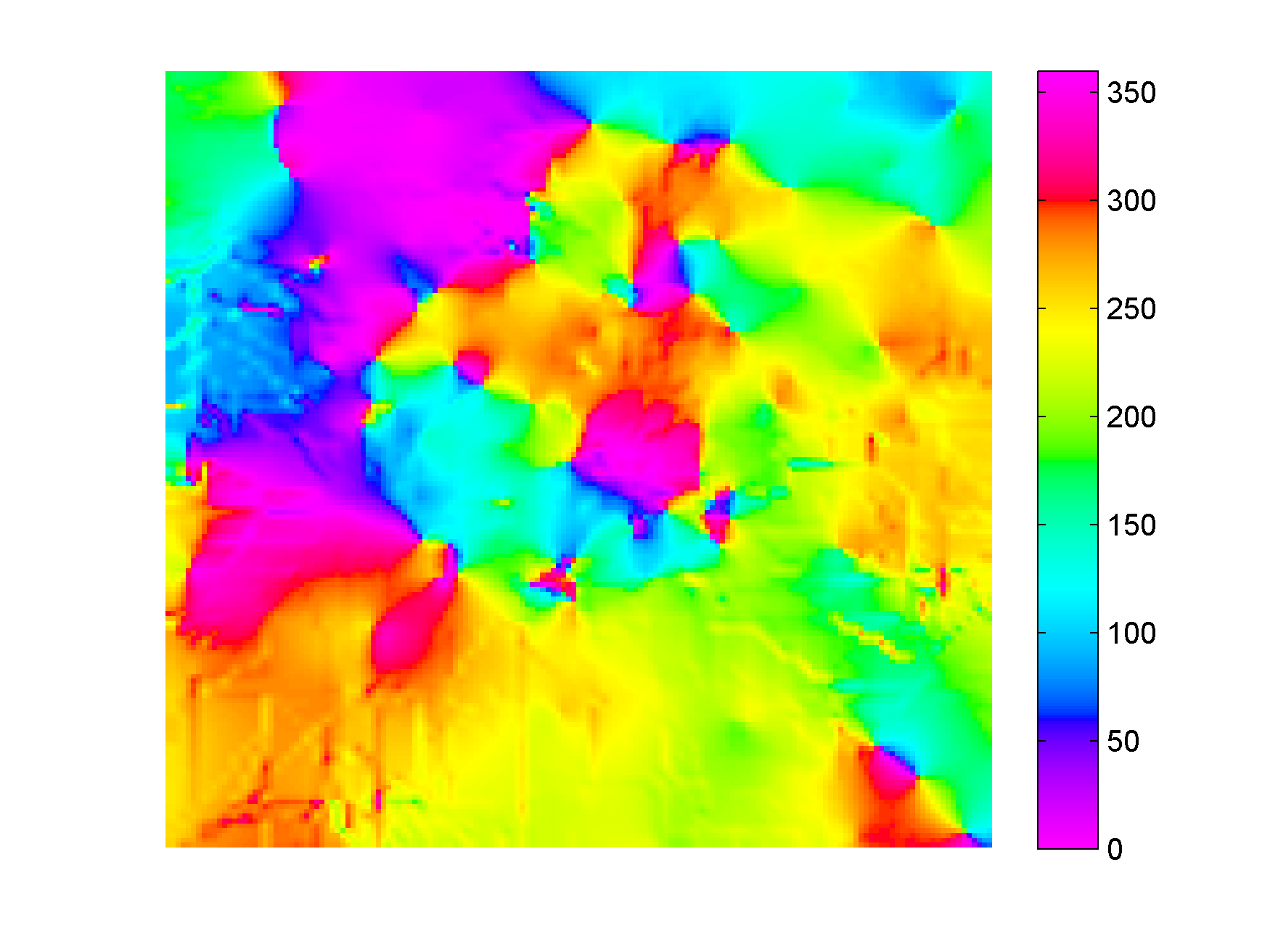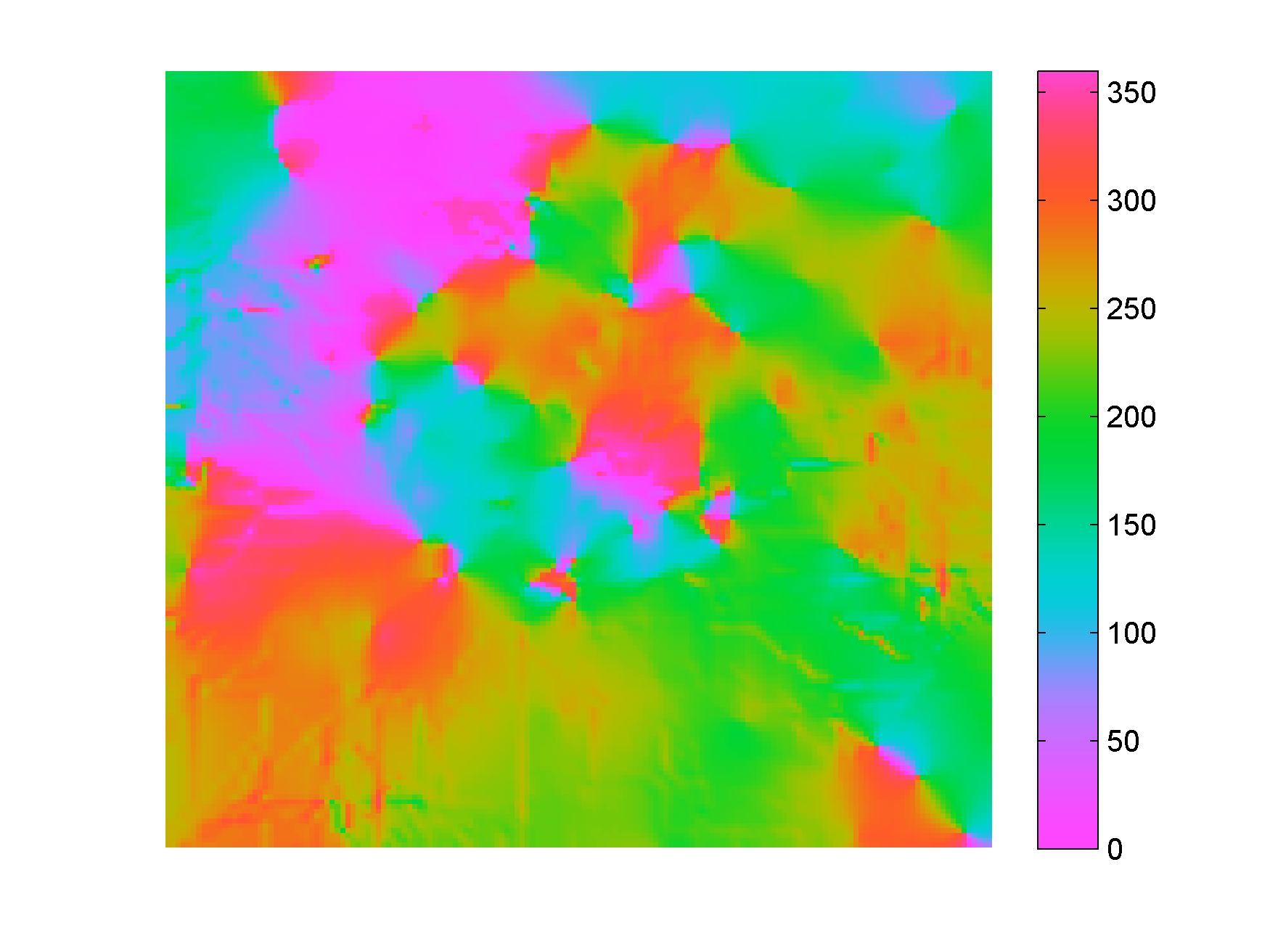# Reinventing the color wheel – part 2

In the first post of this series I argued that we should not build colormaps for azimuth (or phase) data by interpolating linearly between fully saturated hues in RGB or HSL space.

A first step towards the ideal colormap for azimuth data would be to interpolate between isoluminant colours instead. Kindlmann et al. (2002) published isoluminant RGB values for red, yellow, green, cyan, blue, and magenta based on a user study. The code in the next block show how to interpolate between those published colours to get 256-sample R, G, and B arrays (with magenta repeated at both ends), which can then be combined into a isoluminant colormap for azimuth data.

```01 r = np.array([0.718, 0.847, 0.527, 0.000, 0.000, 0.316, 0.718])
02 g = np.array([0.000, 0.057, 0.527, 0.592, 0.559, 0.316, 0.000])
03 b = np.array([0.718, 0.057, 0.000, 0.000, 0.559, 0.991, 0.718])
04 x = np.linspace(0,256,7)
05 xnew = np.arange(256)
06 r256 = np.interp(xnew, x, r)
07 g256 = np.interp(xnew, x, g)
08 b256 = np.interp(xnew, x, b)
```

This is a good example in general of how to interpolate to a finer sampling one or more sequence of values using the interp function from the Numpy library. In line 04 we define 7 evenly spaced points between 0 and 255; this will be the sample coordinate for the r, g, and b colours created in lines 01-03. In line 05 we create the new coordinates we will be interpolating r, g, and b values at in lines 06-08 (all integers between 0 and 256). The full code will come in the Notebook accompanying the last post in this series.

This new colormap is used in the bottom map of the figure below, whereas in the top map we used a conventional HSV azimuth colormap (both maps show the dip azimuth calculated on the Penobscot horizon). The differences are subtle, but with the isoluminant colormap we are guaranteed there are no perceptual artifacts due to the random variations in lightness of the fully saturated HSV colors.Another possible strategy to create a perceptual colormap for azimuth data would be to set lightness and chroma to constant values in LCH space and interpolate between hues. This is the Matlab colormap I previously created, and shown in Figure 4 of New Matlab isoluminant colormap for azimuth data. In the next post, I will show how to do this in Python.

##### Read more on colors and seismic data

The last two posts on Agile show you how to corender seismic amplitude and continuity from a time slice using a 2D colormap,

##### Reference

Kindlmann, E. et al. (2002). Face-based Luminance Matching for Perceptual Colour map Generation – Proceedings of the IEEE conference on Visualization.

# Reinventing the color wheel – part 1

In New Matlab isoluminant colormap for azimuth data I showcased a Matlab colormap that I believe is perceptually superior to the conventional, HSV-based colormaps for azimuth data, in that it does not superimposes on the data the color artifacts that plague all rainbows. However, it still has a limitation, which is that the main colours do not correspond exactly to the four compass directions N, E, W, and S.

My intention with this series is to go back to square one, deconstruct the conventional colormaps for azimuth, and build a new one that has all the desired properties of both perceptual linearity, and correct location of the main colors. All reproducible in Python.

If we wanted to build from scratch a colormap for azimuth (or phase) data the main tasks would be to generate a sequence of distinguishable colours at opposite quadrants, or compass directions (like 0 and 180 degrees, or N and S), and to wrap around the sequence with the same colour at the two ends.

But to do that, we should avoid interpolating linearly between fully saturated hues in RGB or HSL space.

To illustrate why, it is useful to look at the figure below. On the left is a hue circle with primary, secondary, and tertiary colours in a counter-clockwise sequence: red, rose, magenta, violet, blue, azure, cyan, aquamarine, electric green, chartreuse, yellow, and orange. The colour chips are placed at evenly spaced angular distances according to their hue (in radians).

This looks familiar and seems like a natural ordering of colors, so we may be tempted in building a colormap, to just take that sequence, wrap it around at the red (or the magenta) and linearly interpolate to 256 colours to get a continuous colormap , and use it for azimuth data, which is how usually the conventional azimuth colormaps are built.

On the right side in the figure the chips have been rearranged according to their intensity on a counter-clockwise sequence from 0 to 255 with 0 at three hours; so, for example blue, which is the darkest colour with an intensity of 29, is close to the beginning of the sequence, and yellow, the brightest with an intensity of 225, is close to the end. Notice that the chips are no longer equidistant.

The most striking is that the blue and the yellow chips are more separated than the other chips, and for this reason blue and yellow features seem to stand out a lot more in a map when using this color sequence, which can be both distracting and confusing. A good example is Figure 3 in New Matlab isoluminant colormap for azimuth data.

Also, yellow and red, being two chips apart in the left circle in the figure above, are used to colour azimuths 60 degrees apart, and so do cyan and green. However, if we look at the right circle, we realize that the yellow and red chips are much further apart than the cyan and green chips  in the perceptual dimension of intensity; therefore, features colored in yellow and red  could be perceived as much further apart (in azimuth) than cyan and green.

These differences may be subtle, but in my opinion they become important when dip azimuth is combined with other attributes, perhaps using a 3D colormap, and the resulting map is used for detailed structural interpretation. There is a really good example of this type of 3D colormap in Chopra and Marfurt (2007), where dip azimuth is rendered with hue modulation, dip magnitude with saturation modulation, and coherence with lightness modulation.

A code snippet with the main Python commands to generate the two polar scatterplots in the figure is listed, and explained below. The full code can be found in this Jupiter Notebook.

```01 import matplotlib.colors as clr
02 keys=['red', '#FF007F', 'magenta', '#7F00FF', 'blue', '#0080FF','cyan', '#00FF80',
'#00FF00', '#7FFF00', 'yellow', '#FF7F00']
03 my_cmap = clr.ListedColormap(keys)
04 x = np.arange(12)
05 color = my_cmap(x)
06 n = 12
07 theta = 2*np.pi*(np.linspace(0,1,13))
08 r = np.ones(13)*2.5
09 area = 200*r**2 # size of color chips
10 c = plt.scatter(theta, r, c=color, s=area)
11 theta_i = 2*np.pi*(sorted_intensity/255.0)
12 colors = my_sorted_cmap(np.arange(12))
13 c = plt.scatter(theta_i, r, c=colors, s=area)```

In line 01 we import the Colors module from the Matplotlib library, then line 02 creates the desired sequence of colours (red, rose, magenta, violet, blue, azure, cyan, aquamarine, electric green, chartreuse, yellow, and orange) using either the name or Hex code, and line 03 generates the colormap. Then we use lines 04 and 05 to assign colours to the chips in the first scatterplot (left), and lines 06, 07, and 09 to specify the number of chips, the angular distances between chips, and the area of the chips, respectively. Line 10 generates the plot. The modifications in lines 11-14 will result in the scatterplot on the right side in the figure (the sorted intensity is calculated in much the same way as in my Geophysical tutorial – How to evaluate and compare colormaps in Python).

 Or, perhaps, just create 12 discrete colour classes to group azimuth values in bins of pi/6 (30 degrees) each, and wrap around again at the magenta, to generate a discrete colormap.

 The green chip is almost completely covered by the orange chip.

# New Matlab isoluminant colormap for azimuth data

I recently added to my Matlab File Exchange function, Perceptually improved colormaps, a colormap for periodic data like azimuth or phase. I am going to briefly showcase it using data from my degree thesis in geology, which I used before, for example in Visualization tips for geoscientists – Matlab. Figure 1, from that post, shows residual gravity anomalies in milligals.

Often we’re interested in characterizing these anomalies by calculating the direction of maximum dip at each point on the surface, and for that direction display the azimuth, or dip azimuth.  I’ve done this for the surface of residual anomalies from Figure 1 and displayed the azimuth in Figure 2. Azimuth from 0 to 360 degrees are color-coded using Jet, Matlab’s standard colormap (until recently). Typically I do not trust azimuth values when the dip is close to zero because it is often contaminated by noise so I would use shading to de-saturate the colors where dip has the lowest values, but for ease of discussion I haven’t done so in this case.Figure 2. Azimuth values color-coded with Jet.

There are two problems with Figure 2. First, the well-known problems with the jet colormap. For example, blue is too dark and blue areas appear as bands of constant colour. Yellow is much lighter than any other colour so we see artificial yellow edges that are not really present in the data. But there is an additional issue in Figure 2 because azimuths close in value to 0 and 360 degrees are colored with blue and red, respectively, instead of a single color as they should, causing an additional artificial edge.

In Figure 3 I recolored the map using a colormap that replicates those used in many geophysical software tools to display azimuth or phase data. This is better because it wraps around at 360 degrees but the perceptual issues are unresolved: in this case red, yellow and blue all appear as sharp perceptual edges.Figure 3. Azimuth values color-coded with generic azimuth colormap.Figure 4. Azimuth values color-coded with isoluminant azimuth colormap.

In Figure 4 I used my new colormap, called isoAZ (for isoluminant azimuth). This colormap is much better because not only does it wraps around at 360 degrees, but also lightness is held constant for all colors, which eliminates the perceptual anomalies. All the artificial yellow, red, and blue edges are gone, only real edges are left. This can be more easily appreciated in the figure below: if you hover with your mouse over it you are able to switch back and forth between Figure 3 and Figure 4.From an interpretation point of view, azimuths 180 degrees apart are of opposing colours, which is ideal for dip azimuth data because it allows us to easily recognize folds where dips of opposite direction are juxtaposed at an edge. One example is the sharp edge in the northwest quadrant of Figure 4, where magenta is juxtaposed to green. If you look at Figure 1 you see that there’s a relative high in this area (the edge in Figure 4) with dips of opposite direction on either side (East and West, or 0 and 360 degrees).

The colormap was created in the Lightness-Chroma-Hue color space, a polar transform of the Lab color space, where lightness is the vertical axis and at each value of lightness, chroma is the radial coordinate and hue the polar angle. One limitation of this approach is that due to theirregular  shape of the color gamut section at each lightness value, we can never exceed  chroma values  of about 38-40 (at lightness = 65 in Matlab; in Python, with extensive trial and error, I have not been able to go past 36 using the Scikit-image Color module), which make the resulting colors pale, pastely.

it creates For those that want to experiment with it further, I used just a few lines of code similar to the ones below:

```radius = 38; % chroma
theta = linspace(0, 2*pi, 256)'; % hue
L = (ones(1, 256)*65)'; % lightness
Lab = [L, a, b];
RGB=colorspace('RGB<-Lab',Lab(end:-1:1,:));
```

This code is a modification from an example by Steve Eddins on a post on his Matlab Central blog. In Steve’s example the colormap cycles through the hues as lightness increases monotonically (which by the way is an excellent way to generate a perceptual rainbow). In this case lightness is kept constant and hue cycles through the entire 360 degrees and wraps around. Also, instead of using the Image Processing Toolbox, I used  Colorspace, a free function from Matlab File Exchange, for the color space transformations.

For data like fracture orientation where azimuths 180 degrees apart are equivalent it is better to stack two of these isoluminant colormaps in a row. In this way we place opposing colors 90 degrees apart, whereas color 180 degrees apart are the same. You can do it using Matlab commands repmat or vertcat, as below:

```radius = 38; % chroma
theta = linspace(0, 2*pi, 128)'; % hue